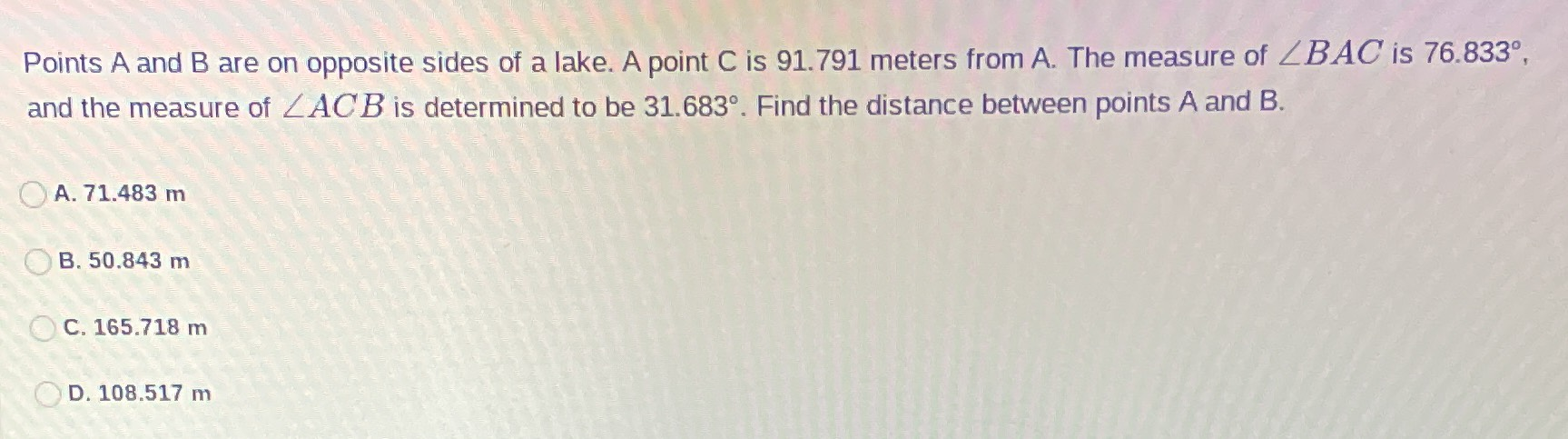### Still have math questions?

Trigonometry
QuestionPoints $$A$$ and $$B$$ are on opposite sides of a lake. A point $$C$$ is $$91.791$$ meters from $$A$$ . The measure of $$\angle B A C$$ is $$76.833 ^ { \circ }$$ , and the measure of $$\angle A C B$$ is determined to be $$31.683 ^ { \circ }$$ . Find the distance between points $$A$$ and $$B$$ .

A. $$71.483 m$$

B. $$50.843 m$$

C. $$165.718 m$$

D. $$108.517 m$$